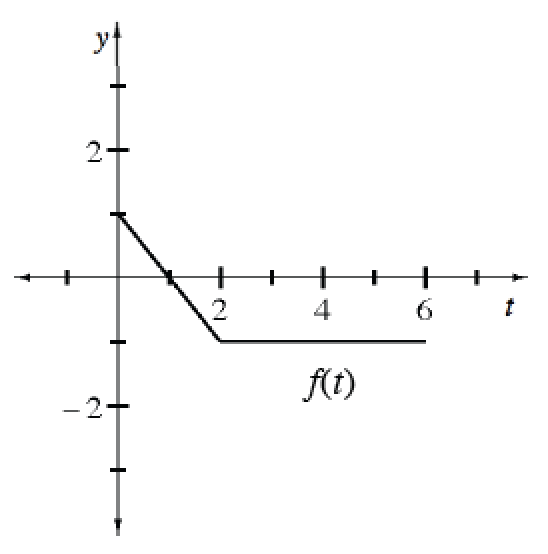### Home > APCALC > Chapter 10 > Lesson 10.1.1 > Problem10-7

10-7.

Examine the graph of $y = f(t)$ shown at right.

Let $F ( x ) = \int _ { 0 } ^ { 2 x - 4 } f ( t ) d t$.

1. What is the domain of $F$?

$f(t)$ is defined for $0 ≤ t ≤ 6$, so $0 ≤ 2x - 4 ≤ 6$.

2. For what values of $x$ does $F$ attain its absolute maximum and minimum? Justify your answer.

$F(x)$ represents the area under the curve. Review the graph.
For what value of $t$ will this area be a maximum? A minimum?

The maximum occurs when $t = 1$. The minimum occurs when $t = 6$.
What are the corresponding $x$-values?